# Square-1 谜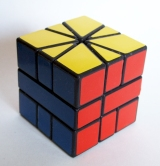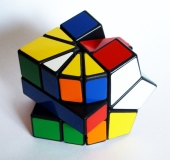Square-1（之前称为 Cube 21 和 Back to Square One）是一个可变形的三层拼图。它的解法非常独特，因为它有风筝形状的角块，边块是三角形的，与拼图的内部机制无法区分。这意味着这些角块可以与边块互换，使得上层有10个块，而下层只有6个。## 如何解决Square-1

### 符号

Square-1的上下两层由细三角形边块组成，而角块的部分较厚且呈风筝形状。一块边块有30º（1步），而一块厚块有60º（2步）宽。在算法中，我们指定需要在切割运动之间旋转上下两层的步数。

/ - 一个切割就像是魔方R层进行180º旋转一样（图像）。Square-1的打乱器有时会忽略切割的动作。
(1, 0) / - 顺时针旋转上层30º并切割
(0, 3) / - 逆时针旋转下层90º并切割。相当于魔方中的D动作。
/ (0, -1) / - 从一个切割开始，逆时针旋转下层30º，然后再切割一次
(2, -1) / - 上层顺时针旋转2步，下层逆时针旋转1步并切割

### 1. 将拼图变成立方体形状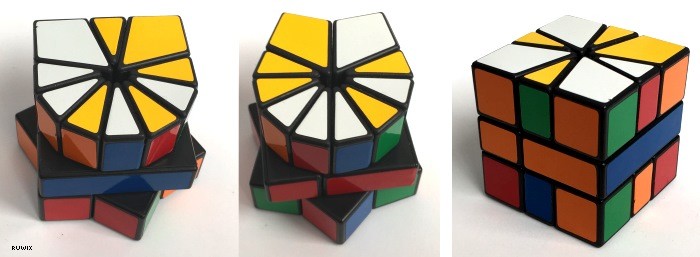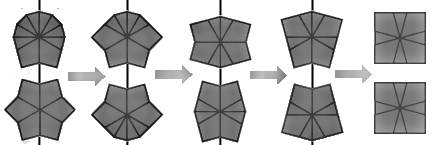### 中心层不正确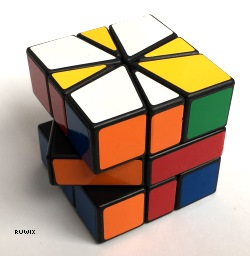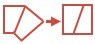如果中间层不是一个正方形，执行以下步骤：(0, -1) / (6, 0) / (6, 0) / (0, 1)

### 2. 顶部角块（黄色）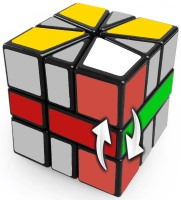(0, -4) / (0, 3) / (0, 1)(1, 0) / (0, -3) / (0, 3) / (0, -3) / (0, -3) / (0, 6) / (-1, 0)

### 3. 边块到它们的层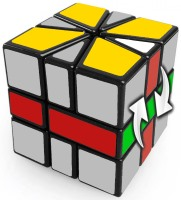(1, 0) / (0, -3) / (0, -3) / (-1, -1) / (1, 4) / (0, 3) / (-1, 0)

### 4. 交换角块/ (3, -3) / (0, 3) / (-3, 0) / (3, 0) / (-3, 0) /

### 5. 排列边块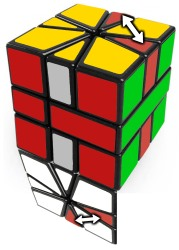(0, 2) / (0, -3) / (1, 1) / (-1, 2) / (0, -2)

### 6. 奇偶性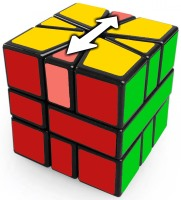/ (3,3) / (1,0) / (-2,-2) / (2,0) / (2,2) / (-1,0) / (-3,-3) / (-2,0) / (3,3) / (3,0) / (-1,-1) / (-3,0) / (1,1) / (-4,-3)

### ¡恭喜，你已经解决了你的Square-1！